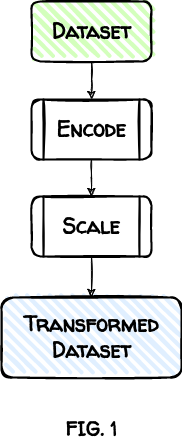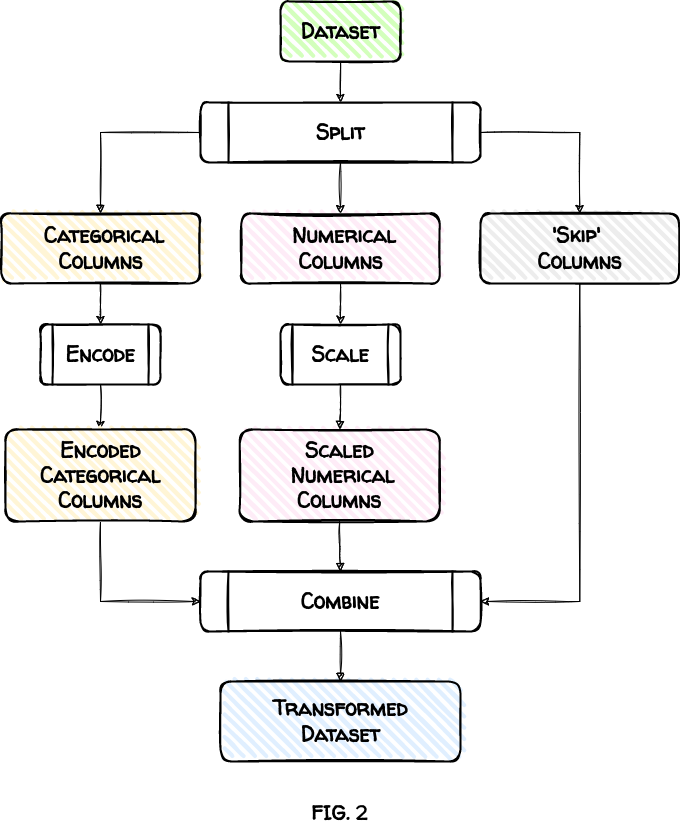# ColumnTransformer: Why and How to Use It to Preprocess Data

Let’s explore why you should always use ColumnTransformer to prepare datasets with different column types.

## Introduction 🔗

The columns in your dataset may need special handling. That’s especially true when you transform them to train a machine learning model.

For example, numerical columns need scaling, and categorical columns should be encoded. And you would want to vectorize columns with text data.

Some columns are fine just the way they are. You don’t want to change them in any way.

How can you apply these different transformations to different types of columns?

We’ll look at three approaches:

1. Apply Transformations Sequentially
2. Split, Transform and Combine
3. Use ColumnTransformer

By the end of this post, you’ll see why you should always use the third approach.

## A Motivating Example 🔗

We’ll use a simplified version of the `titanic` dataset. Here are a few sample rows:

CLASS GENDER AGE FARE RELATIVES SURVIVED
3 male 26.51 7.75 0 0
3 male 40.50 7.75 0 0
1 female 18.00 227.52 1 1
3 female 5.00 12.48 0 1
2 male 32.00 10.50 0 0
1 female 36.00 120.00 3 1
1 female 22.00 66.60 1 1
3 male 25.00 7.22 0 0
1 female 24.00 263.00 5 1
3 female 4.00 16.70 2 1

Let’s have a closer look at the columns:

### Categorical Columns: `CLASS` & `GENDER`🔗

There are only three possible values for passenger’s `CLASS`: 1, 2, and 3. Similarly, the column `GENDER` has only two unique values: male and female.

Both columns contain categorical data and, therefore, should be encoded.

### Numerical Columns: `AGE`, `FARE` & `RELATIVES`🔗

Some machine learning algorithms perform better if numerical inputs have roughly similar mean, standard deviation, and range.

These statistics differ significantly for our numerical columns:

Column Mean Standard Deviation Range
AGE 29.32 13.28 79.58
FARE 32.20 49.69 512.33
RELATIVES 0.90 1.61 10.00

We’ll need to scale these columns. Scaling will set their mean to 0 and standard deviation to 1.

### ‘Skip’ Column: `SURVIVED`🔗

The column `SURVIVED` has only two possible values: 0 and 1. Therefore it’s already in encoded form. We should leave it as is.

In summary, here are the columns and how we want to transform them:

Column(s) Transformation
CLASS, GENDER Encode
AGE, FARE, RELATIVES Scale
SURVIVED Skip

How do we apply these transformations?

Let’s begin with the first of our three approaches.

## 1. Apply Transformations Sequentially 🔗

In this approach, the dataset undergoes a series of transformations, applied one after another.

The output of one transformation becomes the input for the next transformation.

For the `titanic` dataset, we’ll first encode it. Then we’ll take the encoded dataset and scale it.Let’s see this in action. First, we load the dataset:

``````import pandas as pd

titanic.info()
``````
``````<class 'pandas.core.frame.DataFrame'>
RangeIndex: 891 entries, 0 to 890
Data columns (total 6 columns):
#   Column     Non-Null Count  Dtype
---  ------     --------------  -----
0   CLASS      891 non-null    int64
1   GENDER     891 non-null    object
2   AGE        891 non-null    float64
3   FARE       891 non-null    float64
4   RELATIVES  891 non-null    int64
5   SURVIVED   891 non-null    int64
dtypes: float64(2), int64(3), object(1)
memory usage: 41.9+ KB
``````
``````titanic.head()
``````

CLASS GENDER AGE FARE RELATIVES SURVIVED
0 3 male 22.0 7.25 1 0
1 1 female 38.0 71.28 1 1
2 3 female 26.0 7.92 0 1
3 1 female 35.0 53.10 1 1
4 3 male 35.0 8.05 0 0

### 1.1 Apply Encoding 🔗

We encode columns `CLASS` and `GENDER` using pandas' method `get_dummies()`.

It’s standard practice to drop the first level of each encoded categorical column. We’ll do that using the `drop_first` argument.

``````titanic = pd.get_dummies(titanic, columns=['CLASS', 'GENDER'],
drop_first=True)
``````
AGE FARE RELATIVES SURVIVED CLASS_2 CLASS_3 GENDER_male
0 22.0 7.25 1 0 0 1 1
1 38.0 71.28 1 1 0 0 0
2 26.0 7.92 0 1 0 1 0
3 35.0 53.10 1 1 0 0 0
4 35.0 8.05 0 0 0 1 1

The new encoded columns (in green) look good.

### 1.2 Apply Scaling 🔗

Next, we scale using sklearn’s `StandardScaler`:

``````from sklearn.preprocessing import StandardScaler

scaler = StandardScaler()
scaled_titanic = scaler.fit_transform(titanic)

titanic = pd.DataFrame(scaled_titanic, columns=titanic.columns)
``````
AGE FARE RELATIVES SURVIVED CLASS_2 CLASS_3 GENDER_male
0 -0.55 -0.50 0.06 -0.79 -0.51 0.90 0.74
1 0.65 0.79 0.06 1.27 -0.51 -1.11 -1.36
2 -0.25 -0.49 -0.56 1.27 -0.51 0.90 -1.36
3 0.43 0.42 0.06 1.27 -0.51 -1.11 -1.36
4 0.43 -0.49 -0.56 -0.79 -0.51 0.90 0.74

As expected, the numerical columns `AGE`, `FARE`, and `RELATIVES` (in green) are scaled.

But we have a problem. All the other columns were scaled as well.

That’s not what we intended. We had encoded categorical columns in the previous step. But they were scaled in this step and don’t contain 0s and 1s anymore (in yellow).

The column `SURVIVED` (in red) suffered a similar fate.

### 1.3 The Fatal Flaw 🔗

The sequential approach applies every transformation, one after another, to every column.

For example, we wanted to apply only one transformation, encoding, to the column `GENDER`. However, we encoded and then scaled it.

A better technique will ensure that we don’t apply unwanted transformations to any column.

That’s what our second approach will do.

## 2. Split, Transform and Combine 🔗

This approach works in 3 steps:

1. Split the input dataset into subsets based on the column types.

For example, we’ll split the `titanic` dataset into three subsets. The first will have numerical columns. The second will contain categorical columns. The columns we don’t want to transform will go in the third ‘Skip’ subset.

2. Transform each subset based on its column type.

Each subset is independent and only gets the transformations it needs. That means we’ll scale the numerical subset and encode the categorical subset.

3. Combine all the subsets to create our final, transformed dataset.Let’s see these steps in action.

``````titanic = pd.read_csv('titanic.csv')
``````

CLASS GENDER AGE FARE RELATIVES SURVIVED
0 3 male 22.00 7.25 1 0
1 1 female 38.00 71.28 1 1
2 3 female 26.00 7.92 0 1
3 1 female 35.00 53.10 1 1
4 3 male 35.00 8.05 0 0

### 2.1 Split Dataset 🔗

We split the `titanic` dataset into three subsets:

• Categorical columns: `CLASS` and `GENDER`
• Numerical columns: `AGE`, `FARE`, and `RELATIVES`
• ‘Skip’ columns: `SURVIVED`
``````categorical_columns = titanic.loc[:, ['CLASS', 'GENDER'] ]
numerical_columns = titanic.loc[:, ['AGE', 'FARE', 'RELATIVES']]
skip_columns = titanic.loc[:, ['SURVIVED']]
``````

### 2.2 Apply Transformations 🔗

Let’s transform each subset according to its column type.

#### Encode Categorical Columns 🔗

We encode using `OneHotEncoder`:

``````from sklearn.preprocessing import OneHotEncoder

encoder = OneHotEncoder(dtype='int', drop='first')
encoded_raw = encoder.fit_transform(categorical_columns)

categorical_columns = pd.DataFrame(
encoded_raw.toarray(),
columns=encoder.get_feature_names_out()
)
``````

CLASS_2 CLASS_3 GENDER_male
0 0 1 1
1 0 0 0
2 0 1 0
3 0 0 0
4 0 1 1

#### Scale Numerical Columns 🔗

Next, we scale using `StandardScaler`:

``````scaler = StandardScaler()
scaled_raw = scaler.fit_transform(numerical_columns)

numerical_columns = pd.DataFrame(
scaled_raw,
columns=numerical_columns.columns
)
``````

AGE FARE RELATIVES
0 -0.55 -0.50 0.06
1 0.65 0.79 0.06
2 -0.25 -0.49 -0.56
3 0.43 0.42 0.06
4 0.43 -0.49 -0.56

### 2.3 Combine Subsets 🔗

Lastly, we use pandas' method `concat()` to combine the subsets. The argument `axis='columns'` ensures that subsets are stitched horizontally across columns.

``````titanic = pd.concat(
[categorical_columns,
numerical_columns,
skip_columns], axis='columns')

``````

CLASS_2 CLASS_3 GENDER_male AGE FARE RELATIVES SURVIVED
0 0 1 1 -0.55 -0.50 0.06 0
1 0 0 0 0.65 0.79 0.06 1
2 0 1 0 -0.25 -0.49 -0.56 1
3 0 0 0 0.43 0.42 0.06 1
4 0 1 1 0.43 -0.49 -0.56 0

The final dataset looks good. We didn’t change the column `SURVIVED`. Other columns were either encoded or scaled, but not both.

Unlike the sequential approach, we didn’t apply any unintended transformation to any column.

Now, let’s look at `ColumnTransformer`, which does everything we want with virtually no effort.

## 3. Use ColumnTransformer 🔗

We got the desired results using the last approach. But it required a lot of work. We had to split the dataset, transform each subset, and combine all the subsets.

Wouldn’t it be nice if you could list the transformations required for different columns? And the system does all the heavy lifting?

Sklearn’s `ColumnTransformer` is the answer to your prayers.

Let’s see it action. We start by loading the `titanic` dataset:

``````titanic = pd.read_csv('titanic.csv')
``````

CLASS GENDER AGE FARE RELATIVES SURVIVED
0 3 male 22.00 7.25 1 0
1 1 female 38.00 71.28 1 1
2 3 female 26.00 7.92 0 1
3 1 female 35.00 53.10 1 1
4 3 male 35.00 8.05 0 0

To use `ColumnTransformer`, we need to pass the columns and their transformations as a list of tuples. Each tuple should have:

• Name: A unique name.
• Transformer: An instance of a transformer like `OneHotEncoder` or `StandardScaler.` Set it to `passthrough` if you want to skip the columns.
• Columns: The list of columns to transform.

Here’s a quick review of the columns and how we want to transform them:

Column(s) Transformation
CLASS, GENDER Encode
AGE, FARE, RELATIVES Scale
SURVIVED Skip

And here’s the corresponding list of tuples:

``````transformers_list = [
('encode', OneHotEncoder(dtype='int',drop='first'),['CLASS','GENDER']),
('scale', StandardScaler(), ['AGE', 'FARE', 'RELATIVES']),
('skip', 'passthrough', ['SURVIVED'])
]
``````

Next, let’s create an instance of `ColumnTransformer` using the list of tuples:

``````from sklearn.compose import ColumnTransformer

column_transformer = ColumnTransformer(transformers_list)
``````

Then we call the `fit_transform()` method. This one line of code performs all the steps from the previous approach (see FIG. 2).

``````transformed_raw = column_transformer.fit_transform(titanic)
``````

Let’s see the result as a `DataFrame`:

``````titanic = pd.DataFrame(
transformed_raw,
columns=column_transformer.get_feature_names_out()
)
``````

encode__CLASS_2 encode__CLASS_3 encode__GENDER_male scale__AGE scale__FARE scale__RELATIVES skip__SURVIVED
0 0.00 1.00 1.00 -0.55 -0.50 0.06 0.00
1 0.00 0.00 0.00 0.65 0.79 0.06 1.00
2 0.00 1.00 0.00 -0.25 -0.49 -0.56 1.00
3 0.00 0.00 0.00 0.43 0.42 0.06 1.00
4 0.00 1.00 1.00 0.43 -0.49 -0.56 0.00

The final dataset looks good. We transformed (or skipped) every column as expected.

## Conclusion 🔗

We explored three approaches to preparing dataset columns.

The first approach gave us incorrect results. We cannot use it to apply different transformations to different columns.

The second approach isolated the columns and transformed them independently. However, it required us to do the grunt work. We had to write code for the tricky ‘split, transform, combine’ steps.

The final approach, `ColumnTransformer`, performed these steps behind the scenes. It required minimal work and delivered the results we wanted.

There’s one more reason why you should always use `ColumnTransformer`. The other approaches can lead to data leakage, sabotaging your machine learning model.

`ColumnTransformer`, when used with `Pipeline`, can help you fix data leakage. I’ll cover this critical topic in another post.

Title Image by Tama66